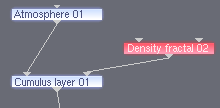### Copy and Paste

Friday, May 16th, 2008 | Author:

Don’t feel like retyping all the shader settings, trying to describe them, or make screen shots?

Then you can simply copy and paste. We know from word processing programs: you select a phrase, hit copy and then keep it on the clipboard. Now this text can be inserted anywhere (as long as the data on the clipboard is not replaced by other data).

``

The same goes for TG2! For example: I want to show someone my settings for clouds. Then I simply select the shader(s) I want, which light up. Via Edit > Copy, or by using [Ctrl]+[C], I copy the nodes and can paste them in forums or elsewhere. For these 3 nodes it will look like this:

```<terragen_clip>
name = "Density fractal 02"
gui_use_node_pos = "1"
gui_node_pos = "200 520 0"
gui_group = "Atmosphere"
enable = "1"
input_node = ""
gui_use_preview_patch_size = "0"
gui_preview_patch_size = "1000 1000"
seed = "41740"
feature_scale = "500"
smallest_scale = "10"
noise_octaves = "13"
apply_high_color = "1"
high_color = "1 1 1"
apply_low_color = "0"
low_color = "0 0 0"
contrast = "1"
roughness = "1.5"
clamp_high = "0"
clamp_low = "0"
apply_displacement = "0"
displacement_direction = "1"
displacement_amplitude = "1"
displacement_offset = "0"
displacement_roughness = "1"
displacement_spike_limit = "1"
continue_spike_limit = "0"
coastline_altitude = "0"
coastline_smoothing = "30"
noise_flavour = "0"
noise_variation = "1"
variation_method = "2"
buoyancy_from_variation = "0.5"
clumping_of_variation = "0.25"
noise_stretch_XYZ = "1 1 1"
distort_by_normal = "0"
distortion_by_normal = "5"
less_warp_at_feature_scale = "1"
allow_vertical_warp = "1"
>
<cloud_layer_v2
name = "Cumulus layer 01"
gui_use_node_pos = "1"
gui_node_pos = "0 460 0"
gui_group = "Atmosphere"
enable = "1"
input_node = "Atmosphere 01"
gui_use_preview_patch_size = "0"
gui_preview_patch_size = "1000 1000"
enable_primary = "1"
enable_secondary = "1"
centre = "4.0886e-022 -6.378e+006 -4.08252e-008"
seed = "0"
cloud_altitude = "2018"
cloud_depth = "500"
edge_sharpness = "5"
cloud_density = "0.006"
cloud_colour = "0.35 0.35 0.35"
scattering_colour = "0.5 0.5 0.5"
sun_glow_amount = "1.5"
sun_glow_power = "0.75"
light_propagation = "2"
light_propagation_mix = "0.125"
fake_internal_scattering = "0.25"
enviro_light = "1"
enviro_light_tint = "1 1 1"
ambient = "0 0 0"
fake_dark_power = "0"
fake_dark_sharpness = "10"
improved_lighting_model = "1"
flatter_base = "0"
invert_profile = "0"
base_wispiness = "0"
base_softness = "0"
coverage_gamma = "1"
rendering_method = "1"
quality = "0.862993"
number_of_samples = "32"
sample_jitter = "1"
acceleration_cache = "2"
>
</cloud_layer_v2>
<planet_atmosphere
name = "Atmosphere 01"
gui_use_node_pos = "1"
gui_node_pos = "0 580 0"
gui_group = "Atmosphere"
enable = "1"
input_node = ""
gui_use_preview_patch_size = "0"
gui_preview_patch_size = "1000 1000"
enable_primary = "1"
enable_secondary = "1"
centre = "-3.8573e-022 -6.378e+006 4.08252e-008"
seed = "0"
haze_density = "4"
haze_horizon_colour = "0.35 0.35 0.35"
bluesky_density = "3.64013"
bluesky_horizon_colour = "0.137358 0.218914 0.274715"
redsky_decay = "3.64013"
haze_exp_height = "2000"
bluesky_exp_height = "8000"
ceiling = "56000"
floor = "-16000"
haze_glow_amount = "1.5"
haze_glow_power = "0.75"
bluesky_glow_amount = "0.25"
bluesky_glow_power = "0.75"
enviro_light = "0.5"
enviro_light_tint = "1 1 1"
ambient = "0 0 0"
fake_dark_power = "0"
fake_dark_sharpness = "10"
bluesky_density_colour = "0.1 0.34 1"
redsky_decay_colour = "0.904837 0.71177 0.367879"
improved_glow_model = "1"
number_of_samples = "64"
sample_jitter = "1"
>
</planet_atmosphere>
</terragen_clip>```

It’s a lot of text, and it looks a bit confusing, but it has the advantage that the recipient of the code can copy the code, and is able to paste it directly into his own node system by using either Edit > Paste or [Ctrl] + [V]!

Besides that, the code isn’t as hard to understand as you might think at first glance – all node parameters are shown in the code. If you want to post only some of the parameters, just copy one node and delete all uninteresting lines of code. Of course, you have to remember that TG2 may not accept that piece of code anymore.

Tags »

Category: Tricks

You can leave a response.

2 Responses

1. 1
Rudolfking

Hi,
I have the problem, that I can’t use the ctrl+c ctrl+v in Terragen 2, neither in text input fields, nor with shaders. Is there any solution? (I use now, the Edit/Copy Edit/Paste, but with many lambert shaders, it’s a bit tiring)

2. 2
Nikita

It seems to be a bug. It works when I start TG2, but after a while, I don’t know how or why, it stops working.